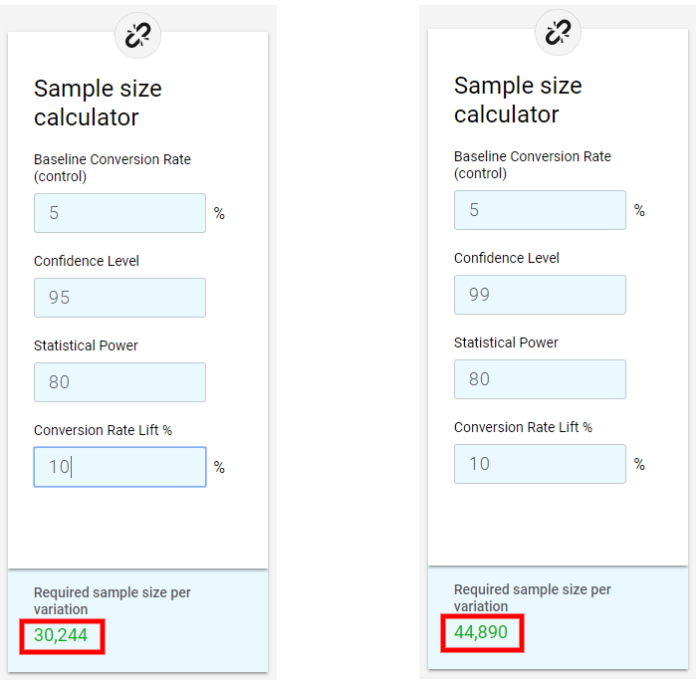Analytics dictionary › P-value

# P-value

P-value is one of the most commonly misunderstood concepts of A/B testing and in statistics in general. Generally, people new to A/B testing tend to believe it describes the probability of a variation being a winner or a loser, compared to the control.

That, of course, is not true. P-value describes a probability of observing the observed (or greater) difference between the control and variation if you assume that your hypothesis is wrong and there should actually be no difference between the groups.

Another way to put this is that p-value describes the probability of seeing the observed difference randomly, say, in an A/A test.

P-value is one of the most important metrics in Frequentist statistics, therefore, it is not about detecting the probability of your null or alternative hypothesis of being true or false nor is it detecting the probability of your variation being better than the control. It is about rejecting or not rejecting the null hypothesis. Here’s how it goes:

1. You come up with some reasonable threshold for rejecting the null hypothesis. The notation used for this threshold is α (the Greek letter alpha). This threshold is a real number between 0 and 1 (usually very close to 0).
2. You promise to yourself in advance that you will reject the null hypothesis if the calculated p-value happens to be below α (and not reject it otherwise).

In A/B testing, the most common threshold α is 0.05, although sites with more traffic that want to minimize the risk of falsely rejecting their null hypothesis often pick 0.01. And sites with little traffic that are looking for quick learnings might go with 0.1.

It is a good practice to wisely choose a suitable threshold and stick to it until there’s a strong enough reason to change it. That way you can determine how many of your null hypothesis were falsely rejected.

I recommend using a statistical significance calculator to see how the chosen threshold affects your needed sample size.